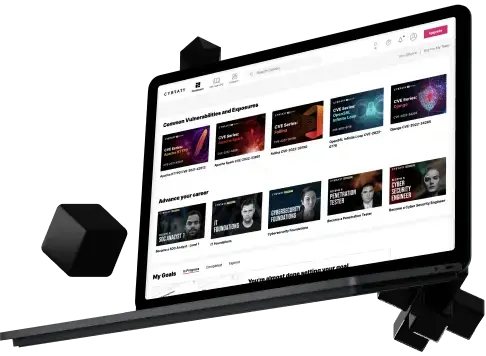# A Quick Mathematical Formula for SubnettingFabien M.Let's begin.Steps:

1. Write powers of 2 left to right
2. Write CIDR subnet masks from 1 to 32. This will take 4 lines, one for each octet in the IP addresses
3. Write the subnet mask: add powers of 2 from left to current column

You can invert 2 and 3 if you think it's more logical (I'm used to this way).You an add the number of usable IP by doing (power of 2) -2A more mathematical way to express the the subnet mask calculation is: current value = value on the left (previous value) + power of 2 of this column.It should take about 1 minute to do this when the exam starts. It will be extremely useful all through the exam to double check any IP and subnet. The only missing part would be a list of possible subnet values (i.e 0, 16, 32, ... for a /28 CIDR), but I found it's best to do this when you need it rather than pre-calculate it. It's not needed that often during the exam, as most of the exam is being "nice" with you and gives you easy values such as 0, 128, 64, ...You should be able to generate this table:2x12864321684211st octet123456782nd9101112131415163rd17181920212223244th2526272829303132mask128192224240248252254255A few examples of how to use this table and concept:- Find the last usable IP in the subnet 172.16.1.0/30/30 gives a 4 total IP's (0 to 3), meaning 2 usable IPs (1 and 2), 0 is the subnet and 3 is the broadcast. Answer is 172.16.1.2- Can 192.168.15.23 use 192.168.16.35 as default gateway if subnet is 255.255.254.0?254 as a mask gives us 2 IP in the third octet, so:

• 192.168.0.x and 192.168.1.x are in the same subnet
• 2.x and 3.x
• 4.x and 5.x
• 6.x and 7.x

The answer is "no," as both IP's are not in the same subnet.- What is the CIDR subnet mask for /12?The answer is 255.240.0.0- What mask do you need to have 64 hosts in a subnet?64 total IP's won't be enough (don't forget broadcast and subnet IP's remove usable IP's from the total). You need to choose a subnet that can accommodate 126 hosts (128-2) hence a mask of 255.255.255.128, or /25Thanks and I hope this is helpful to you.

## Start learning with Cybrary